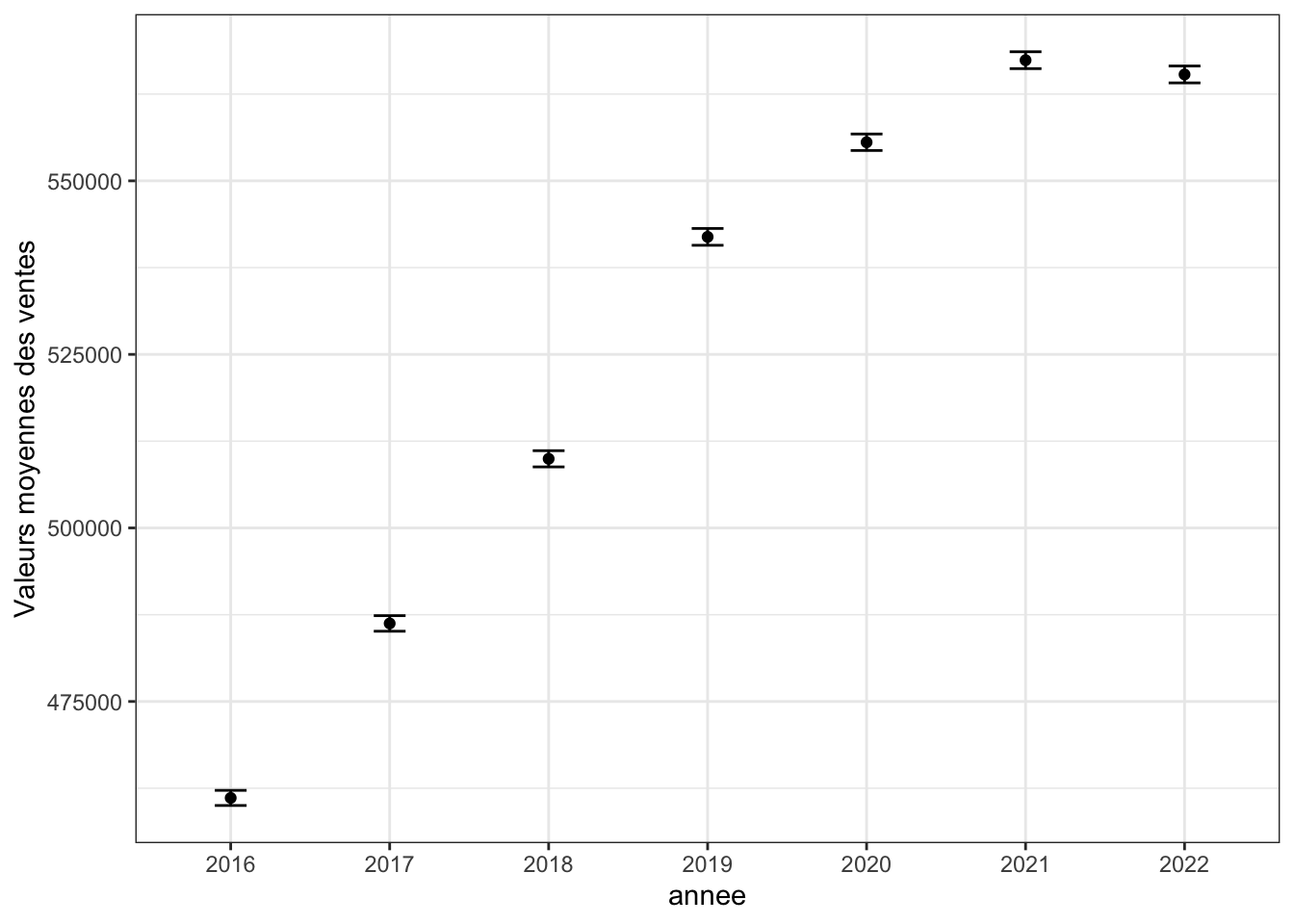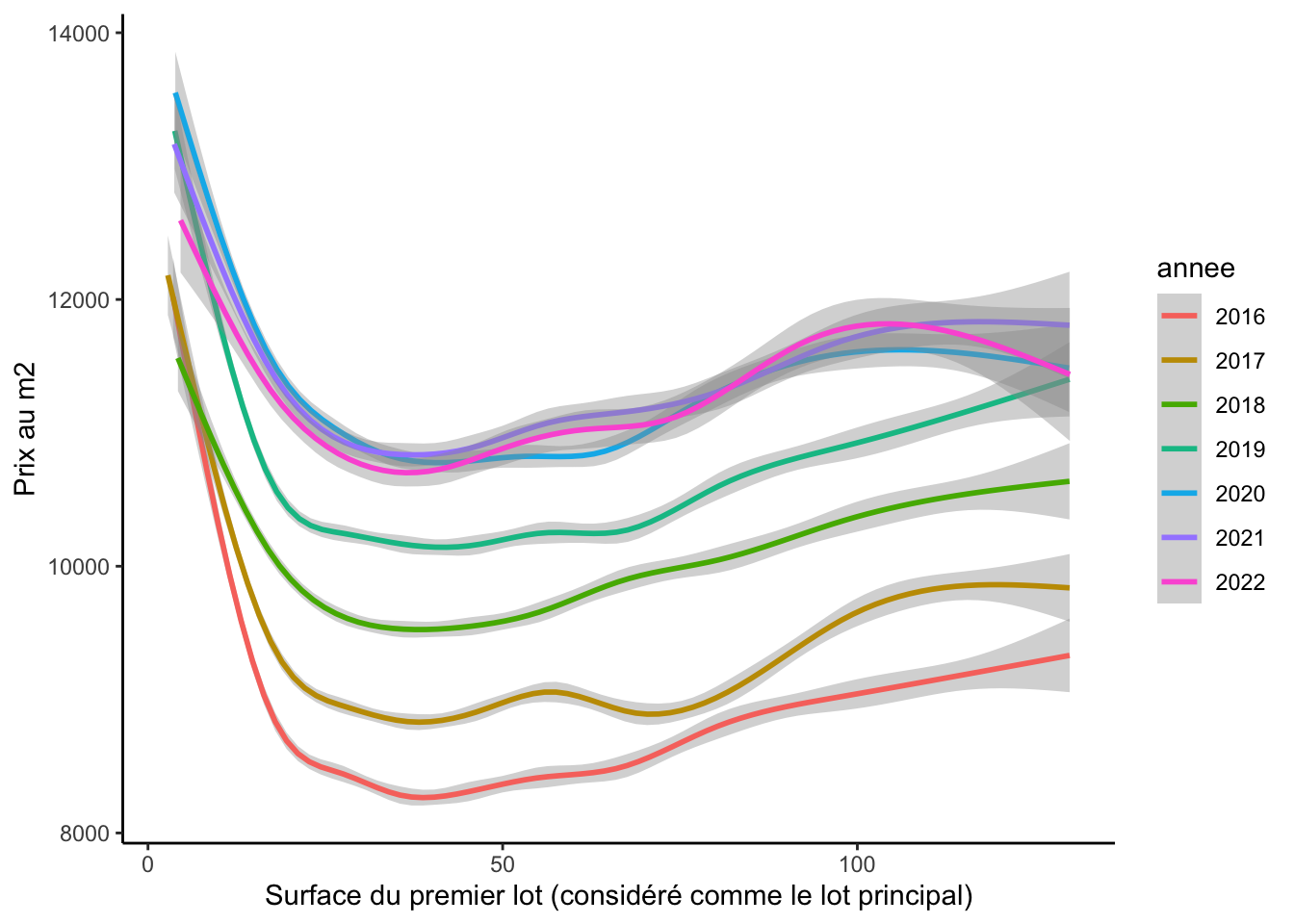# 1 The parisian house market and `data.gouv.fr`

## 1.1 Question 1

This plot is a bit complicated, in particular the confidence intervals.

The different steps are:

• stack the dataframes with `rbind`

• filter a subtable without extreme sales and without the row having missing values in `valeur_fonciere`

• create a colunm year, for this you can use the package `lubridate`, but you can also do it alone with a package extracting the first four characters of a string.

• then use the function `group_by`, to group by year the observations and compute the mean and the standard deviation

• then plot the average over years

• the 95% confidence intervals are obtained from the standard deviation

Again, don’t worry if you don’t succeed to finish everything or understand all the lines. Please note your questions for the next sessions or send an email.

``````knitr::opts_chunk\$set(echo = TRUE)

# We could have done a loop to avoid copy paste, but let's keep it like this for the first class
# Be careful to put the right path (here, I put all the raw data in a folder named 'data')

# handle something strange in 2021
df_2021 <- df_2021[, 2:41]

immo <- rbind(df_2016, df_2017, df_2018, df_2019, df_2020, df_2021, df_2022)

# at the beginning of your notebook
VALEUR_FONCIERE_MAX = 2000000
VALEUR_FONCIERE_MIN = 50000

# Libraries used
library(ggplot2)
library(dplyr) # group_by``````
``````##
## Attaching package: 'dplyr'``````
``````## The following objects are masked from 'package:stats':
##
##     filter, lag``````
``````## The following objects are masked from 'package:base':
##
##     intersect, setdiff, setequal, union``````
``library(lubridate) # function to treat automatically year``
``## Loading required package: timechange``
``````##
## Attaching package: 'lubridate'``````
``````## The following objects are masked from 'package:base':
##
##     date, intersect, setdiff, union``````
``````# Data treatment

## Only keep costal code from Paris
df <- immo[immo\$code_postal %in% c( paste0("7500",1:9),paste0("750",10:20)) ,]

## Define which columns we want to keep
useful_columns <- c("date_mutation",
"valeur_fonciere",
"nature_mutation",
"code_postal",
"nombre_pieces_principales",
#"surface_reelle_bati",
"lot1_surface_carrez",
"type_local",
"nom_commune",
"lot2_surface_carrez",

## take subtable
immo <- immo[immo\$valeur_fonciere < VALEUR_FONCIERE_MAX,]
immo <- immo[immo\$valeur_fonciere > VALEUR_FONCIERE_MIN,]

## create a column year
immo\$annee <- year(immo\$date_mutation)

## remove duplicated lines
nrow(df)``````
``##  728863``
``````df <- unique(df)
nrow(df)``````
``##  619443``
``````## function from package lubridate to get the year and month
immo\$month <- month(immo\$date_mutation, label=TRUE, abbr=FALSE)
immo\$annee <- as.factor(immo\$annee)
# An alternative could be to use `gsub`

# Only keep housing
immo <- immo[immo\$type_local %in% c("Appartement", "Maison"),]

# Only keep transactions
immo <- immo[immo\$nature_mutation == "Vente",]

# Keep only observations for which valeur fonciere is observed
immo <- immo[!is.na(immo\$valeur_fonciere),] # ! indicates "opposite to" so that we keep only rows for which "valeur_fonciere" is observed``````

Check how many transaction by year. Does it seem reasonnable?

``table(immo\$annee)``
``````##
##  2016  2017  2018  2019  2020  2021  2022
## 64927 73959 70247 71531 58540 33657 17979``````
``````summary <- immo  %>%
group_by(annee) %>%
summarise(mean = mean(valeur_fonciere),
sd = sd(valeur_fonciere))

summary\$se = summary\$sd / sqrt(nrow(immo))

ggplot(summary, aes(x = annee, y = mean)) +
geom_point()+
geom_errorbar(aes(ymin=mean-1.96*se, ymax=mean+1.96*se), width=.2,
position=position_dodge(0.05)) +
theme_bw() +
ylab("Valeurs moyennes des ventes")``````💡 In reality, those confidence intervals do not really have an interest as we have access to the whole data set. A confidence intervals reflects the uncertainty, and more precisely the fact that you have sampled a part of an infinite (or way bigger) population. This is clearly not the case here as we have access to the whole transactions. But this is for you to practice.

## 1.2 Question 2

First, we need to do other assumptions

``````## only keep element with surface
immo <- immo[!is.na(immo\$lot1_surface_carrez),]

## Create price per squarre meters: assumptions here on the fact that the lot 1 is the principal one.
immo\$prix.m2 <- immo\$valeur_fonciere / immo\$lot1_surface_carrez``````
``````ggplot(immo[immo\$prix.m2 < 30000 & immo\$lot1_surface_carrez < 130,], aes(x = lot1_surface_carrez, y = prix.m2, group = annee, color = annee)) +
geom_smooth() +
theme_classic() +
ylab("Prix au m2") +
xlab("Surface du premier lot (considéré comme le lot principal)")``````
``## `geom_smooth()` using method = 'gam' and formula = 'y ~ s(x, bs = "cs")'``## 1.3 Question 3

Then, we can observe the number of transactions per month over year to understand what happened in 2020.

``````# 2021 is only first semester so we remove it from the plot
immo[immo\$annee != 2022,] %>%
group_by(annee, month) %>%
summarise(count = n()) %>%
ggplot(aes(x = month, y = count, color = as.factor(annee), group = as.factor(annee))) +
geom_line(size = 1) +
theme_classic() +
theme(axis.text.x = element_text(angle = 90, vjust = 0.5, hjust=1)) +
ylab("Nombre de transactions par mois") +
xlab("")``````
``````## `summarise()` has grouped output by 'annee'. You can override using the
## `.groups` argument.``````
``````## Warning: Using `size` aesthetic for lines was deprecated in ggplot2 3.4.0.